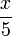# User talk:Emanuele

(Difference between revisions)
 Revision as of 21:04, 14 January 2010 (view source)Emanuele (Talk | contribs) (New page: testing math equations $\frac{x}{2}$ $x^2+3x+5$)← Older edit Revision as of 21:09, 14 January 2010 (view source)Emanuele (Talk | contribs) Newer edit → Line 1: Line 1: testing math equations testing math equations - $\frac{x}{2}$ + $\frac{x}{5}$ $x^2+3x+5$ $x^2+3x+5$

## Revision as of 21:09, 14 January 2010

testing math equations$\frac{x}{5}$

x2 + 3x + 5